Leverages dplyr to process the calculations of a plot inside a database. This package provides helper functions that abstract the work at three levels:

1. Functions that ouput a ggplot2 object
2. Functions that outputs a data.frame object with the calculations
3. Creates the formula needed to calculate bins for a Histogram or a Raster plot

## Installation

You can install the released version from CRAN:

# install.packages("dbplot")

Or the the development version from GitHub, using the remotes package:

# install.packages("remotes")
# remotes::install_github("edgararuiz/dbplot")

## Example

In addition to database connections, the functions work with sparklyr. A local RSQLite database will be used for the examples in this README.

library(DBI)
library(odbc)
library(dplyr)

con <- dbConnect(RSQLite::SQLite(), ":memory:")
db_flights <- copy_to(con, nycflights13::flights, "flights")

## ggplot

### Histogram

By default dbplot_histogram() creates a 30 bin histogram

library(ggplot2)

db_flights %>%
dbplot_histogram(distance)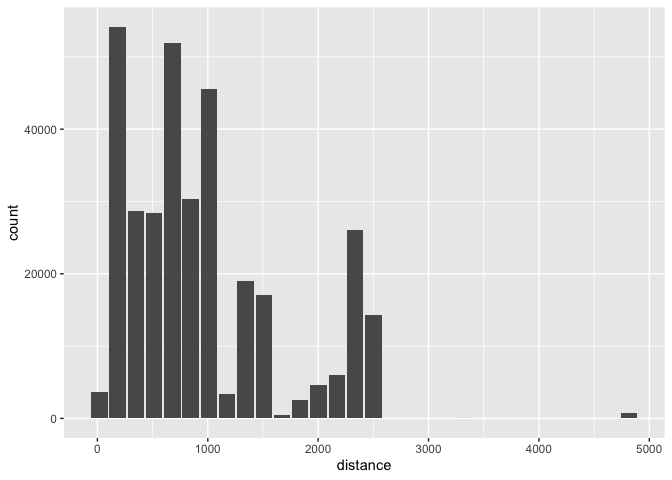Use binwidth to fix the bin size

db_flights %>%
dbplot_histogram(distance, binwidth = 400)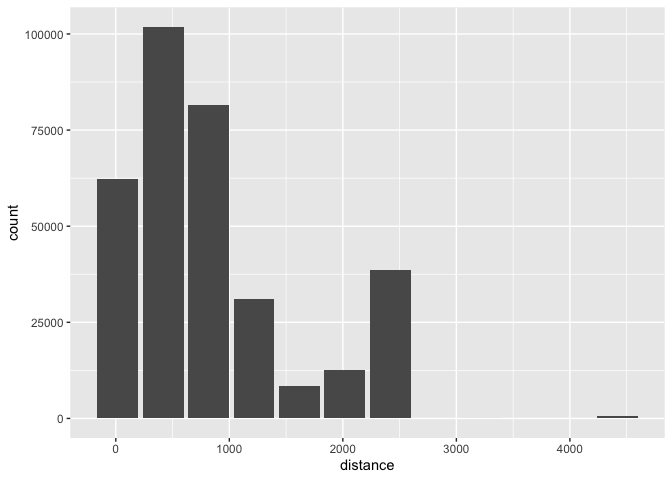Because it outputs a ggplot2 object, more customization can be done

db_flights %>%
dbplot_histogram(distance, binwidth = 400) +
labs(title = "Flights - Distance traveled") +
theme_bw()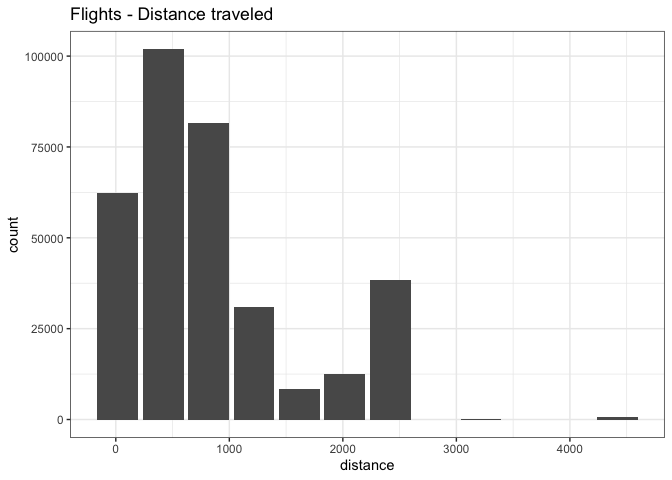### Raster

To visualize two continuous variables, we typically resort to a Scatter plot. However, this may not be practical when visualizing millions or billions of dots representing the intersections of the two variables. A Raster plot may be a better option, because it concentrates the intersections into squares that are easier to parse visually.

A Raster plot basically does the same as a Histogram. It takes two continuous variables and creates discrete 2-dimensional bins represented as squares in the plot. It then determines either the number of rows inside each square or processes some aggregation, like an average.

• If no fill argument is passed, the default calculation will be count, n()
db_flights %>%
dbplot_raster(sched_dep_time, sched_arr_time)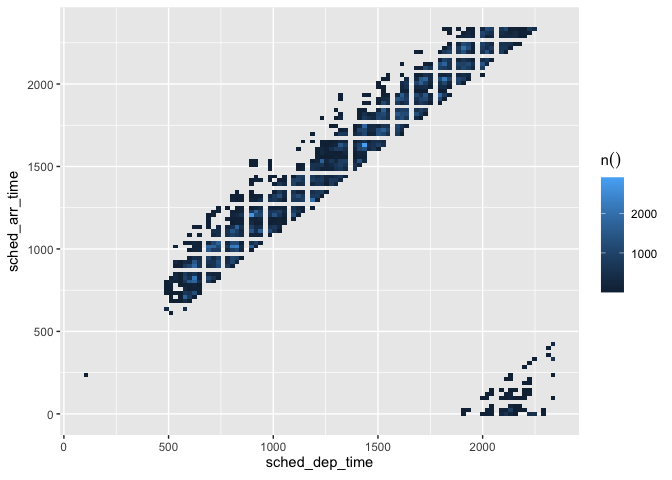• Pass an aggregation formula that can run inside the database
db_flights %>%
dbplot_raster(
sched_dep_time,
sched_arr_time,
mean(distance, na.rm = TRUE)
)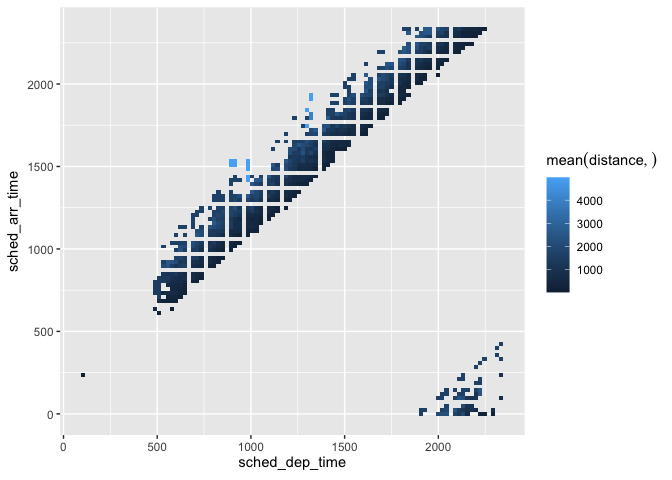• Increase or decrease for more, or less, definition. The resolution argument controls that, it defaults to 100
db_flights %>%
dbplot_raster(
sched_dep_time,
sched_arr_time,
mean(distance, na.rm = TRUE),
resolution = 20
)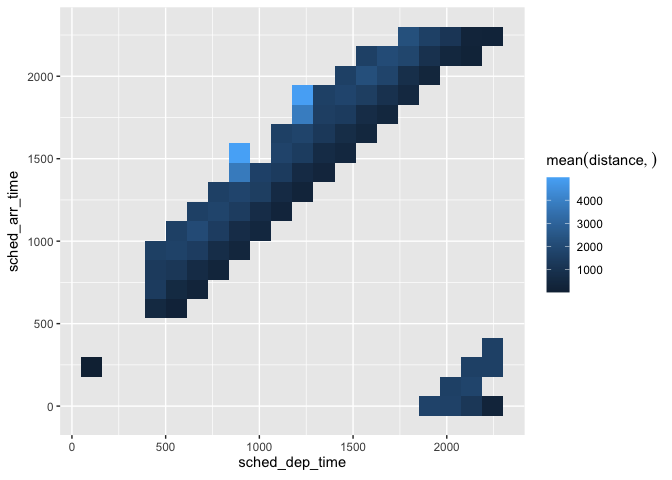### Bar Plot

• dbplot_bar() defaults to a tally() of each value in a discrete variable
db_flights %>%
dbplot_bar(origin)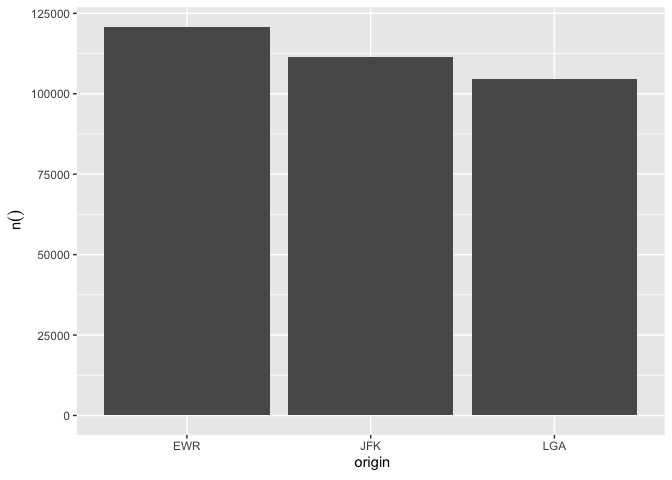• Pass a formula, and column name, that will be operated for each value in the discrete variable
db_flights %>%
dbplot_bar(origin, avg_delay =  mean(dep_delay, na.rm = TRUE))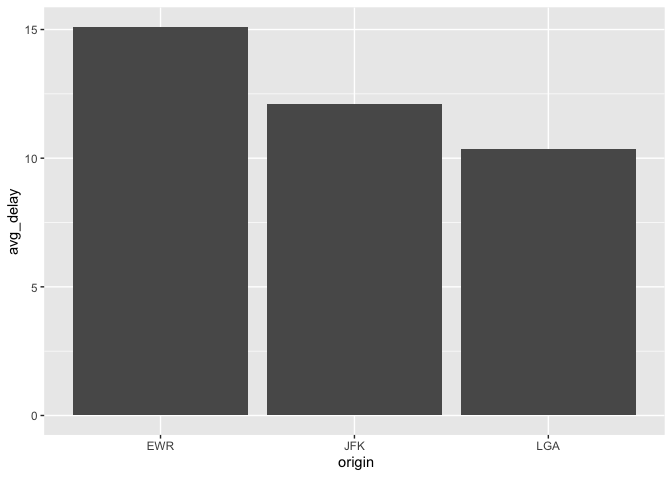### Line plot

• dbplot_line() defaults to a tally() of each value in a discrete variable
db_flights %>%
dbplot_line(month)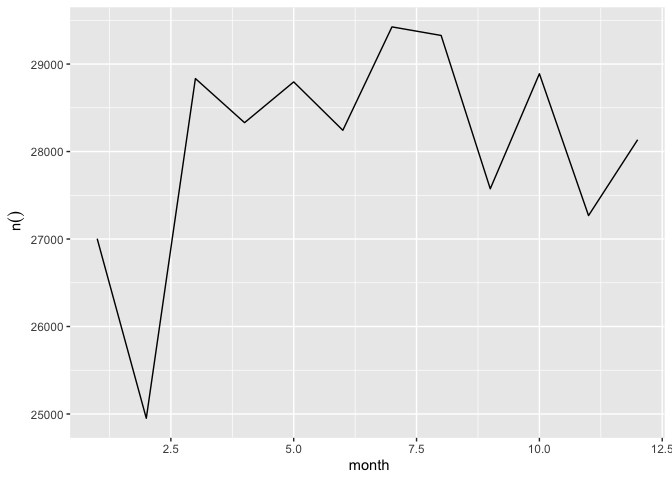• Pass a formula that will be operated for each value in the discrete variable
db_flights %>%
dbplot_line(month, avg_delay = mean(dep_delay, na.rm = TRUE))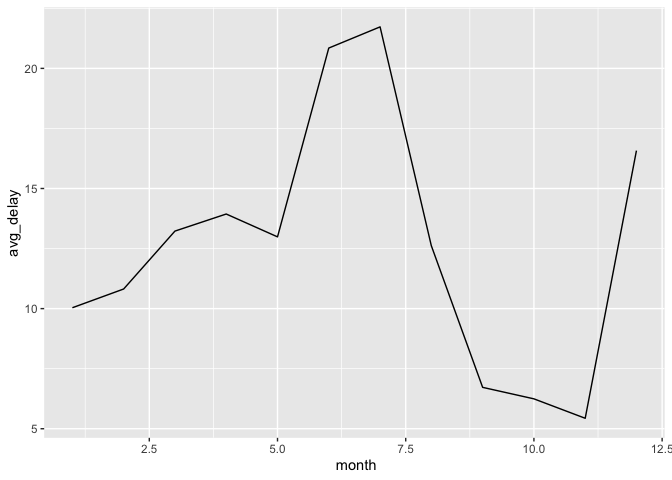### Boxplot

It expects a discrete variable to group by, and a continuous variable to calculate the percentiles and IQR. It doesn’t calculate outliers. It has been tested with the following connections:

• MS SQL Server
• PostgreSQL
• Oracle
• sparklyr

Here is an example using dbplot_boxplot() with a local data frame:

nycflights13::flights %>%
dbplot_boxplot(origin, distance)## Calculation functions

If a more customized plot is needed, the data the underpins the plots can also be accessed:

1. db_compute_bins() - Returns a data frame with the bins and count per bin
2. db_compute_count() - Returns a data frame with the count per discrete value
3. db_compute_raster() - Returns a data frame with the results per x/y intersection
4. db_compute_raster2() - Returns same as db_compute_raster() function plus the coordinates of the x/y boxes
5. db_compute_boxplot() - Returns a data frame with boxplot calculations
db_flights %>%
db_compute_bins(arr_delay)
#> # A tibble: 28 x 2
#>    arr_delay  count
#>        <dbl>  <int>
#>  1     NA      9430
#>  2    -86      5325
#>  3    -40.7  207999
#>  4      4.53  79784
#>  5     49.8   19063
#>  6     95.1    7890
#>  7    140.     3746
#>  8    186.     1742
#>  9    231.      921
#> 10    276.      425
#> # … with 18 more rows

The data can be piped to a plot

db_flights %>%
filter(arr_delay < 100 , arr_delay > -50) %>%
db_compute_bins(arr_delay) %>%
ggplot() +
geom_col(aes(arr_delay, count, fill = count))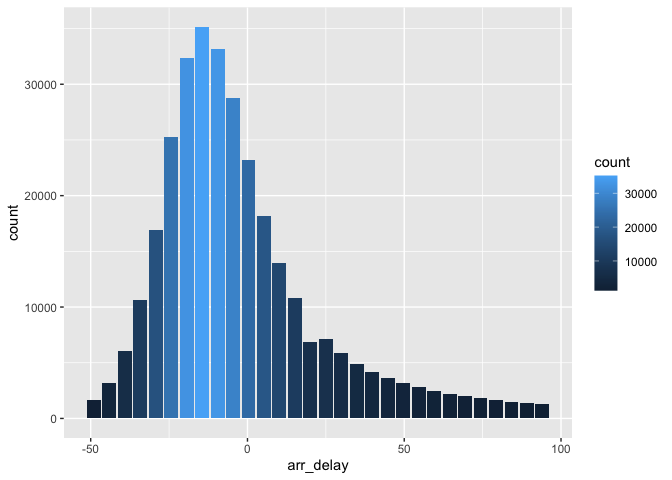## db_bin()

Uses ‘rlang’ to build the formula needed to create the bins of a numeric variable in an un-evaluated fashion. This way, the formula can be then passed inside a dplyr verb.

db_bin(var)
#> (((max(var, na.rm = TRUE) - min(var, na.rm = TRUE))/30) * ifelse(as.integer(floor((var -
#>     min(var, na.rm = TRUE))/((max(var, na.rm = TRUE) - min(var,
#>     na.rm = TRUE))/30))) == 30, as.integer(floor((var - min(var,
#>     na.rm = TRUE))/((max(var, na.rm = TRUE) - min(var, na.rm = TRUE))/30))) -
#>     1, as.integer(floor((var - min(var, na.rm = TRUE))/((max(var,
#>     na.rm = TRUE) - min(var, na.rm = TRUE))/30))))) + min(var,
#>     na.rm = TRUE)
db_flights %>%
group_by(x = !! db_bin(arr_delay)) %>%
tally()
#> # Source:   lazy query [?? x 2]
#> # Database: sqlite 3.29.0 [:memory:]
#>         x      n
#>     <dbl>  <int>
#>  1  NA      9430
#>  2 -86      5325
#>  3 -40.7  207999
#>  4   4.53  79784
#>  5  49.8   19063
#>  6  95.1    7890
#>  7 140.     3746
#>  8 186.     1742
#>  9 231.      921
#> 10 276.      425
#> # … with more rows
db_flights %>%
filter(!is.na(arr_delay)) %>%
group_by(x = !! db_bin(arr_delay)) %>%
tally()%>%
collect %>%
ggplot() +
geom_col(aes(x, n))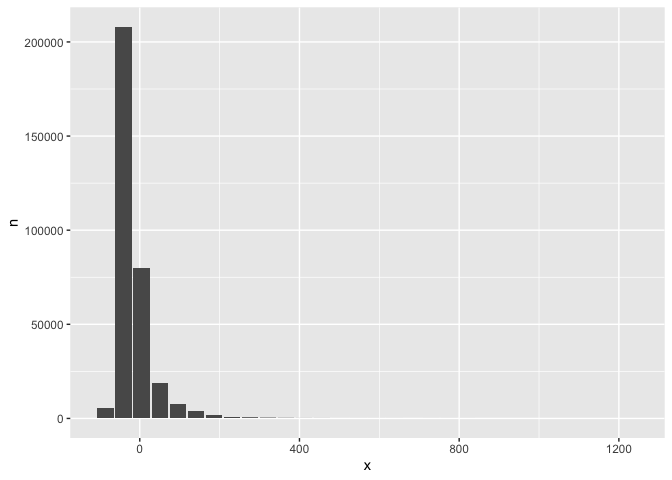dbDisconnect(con)